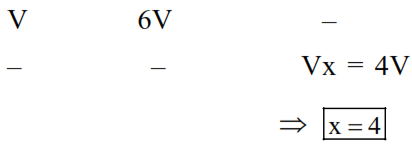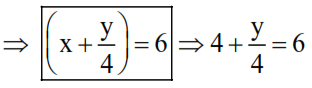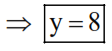Deepak Scored 45->99%ile with Bounce Back Crack Course. You can do it too!

# The formula of a gaseous hydrocarbon which requires 6 times

Question:

The formula of a gaseous hydrocarbon which requires 6 times of its own volume of $\mathrm{O}_{2}$ for complete oxidation and produces 4 times its own volume of $\mathrm{CO}_{2}$ is $\mathrm{C}_{\mathrm{x}} \mathrm{H}_{\mathrm{y}}$. The value of $\mathrm{y}$ is

Solution:

Combustion $\mathrm{rx}^{\mathrm{n}}$ :

$\mathrm{C}_{\mathrm{x}} \mathrm{H}_{\mathrm{y}(\mathrm{g})}+\left(\mathrm{x}+\frac{\mathrm{y}}{4}\right) \mathrm{O}_{2}(\mathrm{~g}) \rightarrow \mathrm{x} \mathrm{CO}_{2}(\mathrm{~g})+\frac{\mathrm{y}}{2} \mathrm{H}_{2} \mathrm{O}(\ell)$Sinc : (I) $\mathrm{Vo}_{2}=6 \times \mathrm{V}_{\mathrm{C}_{\mathrm{x}} \mathrm{H}_{\mathrm{y}}}$

$\Rightarrow \mathrm{V}\left(\mathrm{x}+\frac{\mathrm{y}}{4}\right)=6 \mathrm{~V}$